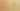ComparisonWithBoolLiteral

Misconception:

To determine whether an expression evaluates to True or False, one must use a relational operator (== or !=) to compare with a bool literal (True or False)Incorrect

To test whether an expression is True or False, one must compare it to True or to False

Correct

To test whether an expression is True or False, one can just use it

CorrectionHere is what's right.

Such a comparison is unnecessary

if CONDITION == True:
...

This can be simplified to

if CONDITION:
...

This applies to all uses of equality operators on bool objects

ExpressionEquivalent Simplified Expression
e == Truee
e == False!e
e != True!e
e != Falsee

Argumentation

One may want to ask a student with this misconception which of the following two code snippets they prefer, and why:

print(age + 0)
print(age)

Then one can ask if they prefer the following:

print(stop == True)
print(stop)

Finally, one can discuss what just writing age — and just writing stop — actually means. One may also want to ask a student whether they would ever say “I am hungry is true” instead of just saying “I am hungry”. In a way, the former could be seen as a low-level statement, where one is concerned about “hungry” being a variable, while the latter is a higher-level statement, where “hungry” just means what it usually means. While in our experience the above arguments do not always lead to a change in practice or attitude (i.e., students might continue to write explicit comparisons), we believe they help to provide a deeper understanding of expressions.

OriginWhere could this misconception come from?

This misconception may go back to textbooks and instructors using the term condition to describe boolean expressions in conditional statements and loops.

if CONDITION:
...
while CONDITION:
...

Students may thus assume that a condition is a separate syntactic construct and is different from a boolean expression. This may include assumptions that a condition must include a comparison, or that it must test whether a variable contains a specific value, or even that only a small number of very specific conditions are possible (i.e., that conditions cannot be arbitrarily composed the way expressions can be).

SymptomsHow do you know your students might have this misconception?

We observed two kinds of symptoms: specific patterns in code, and specific explanations.

Code Patterns

In the following examples, assume that CONDITION is some boolean expression (e.g., is_happy, i < length, pacman.is_hungry(), or a and b).

if CONDITION == True:
...
return CONDITION == True

Explanation

One explanation we observed was that a condition like stop == True was considered to be more explicit, and thus more easily understandable, than the equivalent condition stop. The reason given was that stop == True would show that the program would go check whether the variable stop contained the value True, while the expression stop would not be as descriptive.

ValueHow can you build on this misconception?

This misconception provides an opportunity to discuss that conditions are a type of expressions. Moreover, it provides an opportunity to a discuss the power of composition, and how expressions can be composed of all kinds of subexpressions.

Python